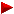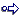HOME plateWON | World!OfNumbersMore Palindromic Productsof Integer SequencesSum of First NumbertypesReversal ProductsPythagorean TriplesPalindromes in other BasesPalindromes in ConcatenationsVarious Palindromic Sums

Introduction

Palindromic numbers are numbers which read the same fromleft to right (forwards) as from the right to left (backwards)Here are a few random examples : 525, 3883, 946101649

Palindromic Products of Integers Sequences are defined and calculated by this extraordinary intricate and excruciatingly complex formula.
So, this line is for experts only(S1) x (S2) x (S3) x ...
As you can see from a quick glance at this page, many sequences just took a fresh start.
So, there is your chance to contribute. You may add to existing topics or you may even send
in totally new topics. It's up to you!

More Palindromic Products of Integer Sequences

Allow me to show you one of my most favourite palindromic numbers323323
Why this number ?
Well, mainly because it can be expressed as the result of some remarkable and unrelated operations.323323 7 x 11 x 13 x 17 x 19 17 + 22 + 38 + 49 + 55 + 66 + 71 + 84 + 93 323323 is a substring of its base 4 representation 1032{323323}. The string 323323 was found at position 985589 counting from the first digit after the decimal point of. [ Pi-Search Page ]

The first is as the product of  Five consecutive primes !
The second as a sum of the nine digits 1 through 9 [ 123456789 ] each raised to a power.
The different exponents thereby forming a combination of these same nine digits ! [ 728956143 ]
The third and the fourth are self-explanatory...
Furthermore by adding the squares of the digits of my palindrome we find :
32 + 22 + 32 + 32 + 22 + 32 = 44
The left or right half of my palindrome is also a palindrome : 323

The product of these Two consecutive palindromes
11 x 22 equals 242.
242 also is the sum of four consecutive integers namely
59 + 60 + 61 + 62
and is also the sum of four consecutive palindromes :
44 + 55 + 66 + 77

Enjoyable palindromic outcomes with Threefold sequence (n) x (n+2) x (n+4)

35 x 37 x 39 = 50505
35 + 37 + 39 = 111
Here's a bonus operation : 50505 / 111 = 455

Find two numbers which differ by 22 so that when each number x is multiplied with (x+1)
they form a palindromic number pair.
Answer by Salil Palkar & Nishant Redkar, Mumbai, India:

Regarding the problem of the palindromic number pair, the
two numbers are 5291 and 5313. This is how me and my
friend cracked it: To have x(x+1) as a palindrome number x
must have 1, 2, 3, 6, 7 or 8 in the units place. Since the two
numbers differ by 22, units place digit should differ by 2 — ie,
it can be 3 and 1 in the unit places of the two numbers or 8
and 6. We find for x(x+1) we have 2 in the units place for
numbers ending with 3, 1, 6, 8. Now a palindrome has 2 in
the units place if it starts with 2. Also since the first digit of
the palindrome is 2 then first digit of x has to be 1, 4 or 5. On
trying further there are 32 pairs possible but on trying on the
calculator we were left with only 16 pairs for 4 or 3 digit
numbers. On analysing further we were left with only one
pair and it is 5291 and 5313. For x = 5291, x(x+1) =
27999972 and for x = 5313, x(x+1) = 28233282.

A Curious Pair of Consecutive Primes

1051 & 1061

Have you noticed the following with Two consecutive primes

1051 x 1061 = 1115111
1051 + 1061 = 2112
10512 + 10612 = 2230322
All three operations yield a palindromic number !
Note that we can easily transform these numbers into palindromic primes by simply inserting a zero.
1051 and 1061 becomes 10501 and 10601 respectively.
Both these numbers can be expressed as the sum of three consecutive primes :
10501 = 3491 + 3499 + 3511
10601 = 3529 + 3533 + 3539
Inserting one more zero makes another interesting product, Hugo Sánchez  reported
from Caracas Venezuela [ March 17, 1999 ].
100501 x 100601 = 10110501101 ( ps.: 100601 is composite.)
Hugo played around with the primes 1051 and 1061 and found more palindromes by inserting various operation signs.
(1051) + (10x61) = 1661
(10^5+1) x (10^6+1) = 100001100001

The two consecutive primes 1051 and 1061 share the following property (Sloane A052033).
All the base b representations (b < 10) with expansions interpreted as decimal numbers are composite.

 1051 10 1387 9 = 19 * 73 2033 8 = 19 * 107 3031 7 = 7 * 433 4511 6 = 13 * 347 13201 5 = 43 * 307 100123 4 = 59 * 1697 1102221 3 = 3 * 3 * 3 * 40823 10000011011 2 = 19 * 20771 * 25339 1061 10 1408 9 = 2 * 2 * 2 * 2 * 2 * 2 * 2 * 11 2045 8 = 5 * 409 3044 7 = 2 * 2 * 761 4525 6 = 5 * 5 * 181 13221 5 = 3 * 3 * 13 * 113 100211 4 = 23 * 4357 1110022 3 = 2 * 199 * 2789 10000100101 2 = 613 * 4027 * 4051
If 1049 is included the property holds also for the three consecutive primes 1049, 1051 and 1061 !
See Prime Curios! 1049

[ July 16, 2001 ]
If we are to find the smallest prime to be appended to 1051 or 1061 so that
the concatenation is a square then the following beautiful solutions pop up :
1051 & 8758721 yielding the square 10518758721.
Note that this square is 1025612

The real  gem  comes with prime 1061 though !
1061 & 10601 yielding the square 106110601.
The appended prime is the same as the original prime with only an extra zero inserted.
Note that this square is 103012 and that 10601 and 10301 are both palindromic primes !

10512 equals 5! + 0! + 6! + 9! + 7! + 7! + 9! + 7! + 9!
Both 1051 and 506977979 are prime !
Source : Prime Curios! 506977979 by G. L. Honaker, Jr.

```

```

The Table

Index NrBase Integer SequenceLength
Palindromic Product of Integer SequencesLength

Fivefold sequence (n) x (n+2) x (n+4) x (n+6) x (n+8)
Searched up to basenumber 100.000.000
1 31 x 33 x 35 x 37 x 392
51.666.6158

Five consecutive primes
1 7 x 11 x 13 x 17 x 191 - 2
323.3236

Fourfold sequence (n) x (n+2) x (n+4) x (n+6)
Searched up to basenumber 100.000.000
2 12 x 14 x 16 x 182
48.3845
1 7 x 9 x 11 x 131 - 2
9.0094

Four consecutive primes
1 5 x 7 x 11 x 131 - 2
5.0054

Three consecutive palindromic primes
1 7 x 11 x 1011 - 2 - 3
7.7774
0 [1 is not considered as a prime] 1 x 2 x 31
61

Three consecutive palindromes
Note that all palindromes (except the first one) can be constructed by using pattern expansion.
Example of one infinite pattern by repeatedly inserting more zero's :
1356531
1030506050301
1003005006005003001 etc.
The length of the numbers is the sequence 7, 13, 19, 25, ... [each time +6].
Make a sequence starting with group (1,3,4).
Apply now to each term the operation (+6,+6,+6) and repeat the process with the new group.
We get : 1,3,4, 7,9,10, 13,15,16, 19,21,22, 25,27,28, 31,33,34, ... (Sloane's A029739)
These sequential numbers starting from the second group match exactly the number of digits
of the following palindromes which are the product of three consecutive palindromes !
13 1.000.000.001 x 1.000.110.001 x 1.000.220.00110
1.000.330.027.200.660.027.200.330.00128
12 999.979.999 x 999.989.999 x 999.999.9999
999.969.997.200.060.002.799.969.99927
11 100.000.001 x 100.010.001 x 100.020.0019
1.000.300.050.006.000.500.030.00125
10 10.000.001 x 10.011.001 x 10.022.0018
1.003.302.720.660.272.033.00122
9 9.997.999 x 9.998.999 x 9.999.9997
999.699.720.060.027.996.99921
8 1.000.001 x 1.001.001 x 1.002.0017
1.003.005.006.005.003.00119
7 100.001 x 101.101 x 102.2016
1.033.272.662.723.30116
6 99.799 x 99.899 x 99.9995
996.972.060.279.69915
5 10.001 x 10.101 x 10.2015
1.030.506.050.30113
4 1.001 x 1.111 x 1.2214
1.357.887.53110
3 979 x 989 x 9993
967.262.7699
2 101 x 111 x 1213
1.356.5317
1 1 x 2 x 32
61

Three consecutive primes
1 7 x 11 x 131 - 2
1.0014

Threefold sequence (n) x (n+2) x (n+4)
Searched up to basenumber 100.000.000
2 202 x 204 x 2063
8.488.8487
1 35 x 37 x 392
50.5055

Three consecutives (n) x (n+1) x (n+2)
Searched up to basenumber 100.000.000
2 77 x 78 x 792
474.4746
1 1 x 2 x 31
61
Record 7 found by Jan van Delden on [ May 20, 2011 ] (source)
Record 6 found by PDG on [ May 9, 1997 ]

Two consecutive primes
Searched exhaustively up to length 20.
One can also find these rare numbers in Sloane's table :
%N Palindromes that are product of two consecutive primes. under A028979.
%N Product of prime and following prime is palindromic. under A028888.
%N Product of prime and previous prime is palindromic. under A028978.
7 13.422.495.703 x 13.422.495.72711
180.163.391.219.193.361.08121
6 Prime Curios!    1.934.063 x 1.934.0717
3.740.615.160.47313
5 1.051 x 1.0614
1.115.1117
4 191 x 1933
36.8635
3 17 x 192
3233
2 7 x 111 - 2
772
1 2 x 31
61

Two consecutive palindromic primes
7 111.010.111 x 111.020.1119
12.324.354.845.342.32117
6 100.111.001 x 100.131.0019
10.024.214.741.242.00117
5 100.060.001 x 100.111.0019
10.017.106.860.171.00117
4 101 x 1313
13.2315
3 11 x 1012 - 3
1.1114
2 7 x 111 - 2
772
1 2 x 31
61
0 [1 is not considered as a prime] 1 x 21
21

Two consecutives (n) x (n+1)

Two consecutive palindromes
This rather boring series is very easy to continue...
I stopped with 1111000001111 x 1111001001111
which gives 1234322113468643112234321
11 999 x 1.0013 - 4
999.9996
10 202 x 2123
42.8245
9 121 x 1313
15.8515
8 111 x 1213
13.4315
7 101 x 1113
11.2115
6 99 x 1012 - 3
9.9994
5 77 x 882
6.7764
4 11 x 222
2423
3 9 x 111 - 2
992
2 2 x 31
61
1 1 x 21
21

Twofold sequence (n) x (n+2)

Contributions

Hugo Sánchez (email) found some interesting palindromes - go to topic.

```

```

[TOP OF PAGE]

Patrick De Geest - Belgium- Short Bio - Some Pictures